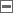# Arsip Kategori: Komputer

## mysql_num_rows

>

(PHP 4, PHP 5)
mysql_fetch_rowGet a result row as an enumerated array

###Description

array mysql_fetch_row ( resource \$result )
Returns a numerical array that corresponds to the fetched row and moves the internal data pointer ahead.

###Parameters

result
The result resource that is being evaluated. This result comes from a call to mysql_query().

###Return Values

Returns an numerical array of strings that corresponds to the fetched row, or FALSE if there are no more rows.
mysql_fetch_row() fetches one row of data from the result associated with the specified result identifier. The row is returned as an array. Each result column is stored in an array offset, starting at offset 0.

###Examples

Example #1 Fetching one row with mysql_fetch_row()

``` <?php\$result = mysql_query("SELECT id,email FROM people WHERE id = '42'");if (!\$result) {    echo 'Could not run query: ' . mysql_error();    exit;}\$row = mysql_fetch_row(\$result); ```

`echo \$row; // 42echo \$row; // the email value?> `

###Notes

Note: This function sets NULL fields to the PHP NULL value.

###See Also

jhulbert at redf dot com
19-Feb-2010 09:32

``` Creates table from all db info: <?php\$qry = "SELECT * FROM exp_member_data";\$res = mysql_query(\$mem_qry); function mysql_fetch_all(\$res) {   while(\$row=mysql_fetch_array(\$res)) {       \$return[] = \$row;   }   return \$return;} function create_table(\$dataArr) {    echo "<tr>";    for(\$j = 0; \$j < count(\$dataArr); \$j++) {        echo "<td>".\$dataArr[\$j]."</td>";    }    echo "</tr>";} \$all = mysql_fetch_all(\$res); echo "<table class='data_table'>"; for(\$i = 0; \$i < count(\$all); \$i++) {    create_table(\$all[\$i]);} echo "</table>"; ```

`?> `

larkitetto at gmail dot com
21-Feb-2008 11:29

``` sry :) note now fixed: ```

`<?php\$esi=mysql_list_tables(\$db);\$ris=mysql_fetch_row(\$esi);//example: \$db has >= 1 tabsecho var_dump(\$ris);//echoes only array(1). solution:while(\$ris=mysql_fetch_row(\$esi)) echo \$ris;/*debug:\$ris=array("1st_tab"); ... \$ris=array("n_tab");\$ris=false;*/while (\$ris[]=mysql_fetch_row(\$esi));//debug:\$ris=array(array("1st_tab"), ... array("n_tab"));echo \$ris[n];//echo:"n_tab"echo \$ris[n];//echo:array | null?>hope it helps `

ryhan_balboa at yahoo dot com
16-Nov-2007 05:23

``` The following are the basic codes to get a specific row from the mysql db into a \$row variable: \$query = "SELECT * FROM table"; \$result = mysql_query(\$query); \$row = mysql_fetch_row(\$result); And \$row, \$row ... \$row[n] are used to access those field values. Does anyone know how I can add a new field to \$row, so that the field count increases from n to n+1? I have tried treating \$row like an array, and tried array_push function, but didn't work. ```

`Thanks. `

m dot s at programmers-online dot net
17-Nov-2005 09:56

``` The following function to read all data out of a mysql-resultset, is may be faster than Rafaels solution: ```

`<?function mysql_fetch_all(\$result) {   while(\$row=mysql_fetch_array(\$result)) {       \$return[] = \$row;   }   return \$return;}?> `

mysql at polyzing dot com
12-Jul-2003 10:05

``` It is probably worth pointing out that the array elements will actually be of type string, OR NULL if the field is null in the database. Thus, either use a double equal comparison to look for empty or null Or, use a triple equal comparison to be able to distinguish the two cases e.g. if (\$field === '') echo "Empty, not NULL\n"; if (\$field === NULL) echo "NULL\n"; ```

`if (\$field == '') echo "Empty or NULL\n"; `

michael and then an at sign wassupy.com
08-Apr-2003 07:09

``` to print an array, simply use print_r(array name) like this:    \$myrow = mysql_fetch_row(\$result);echo "<pre>";print_r(\$myrow);echo "</pre>"; ```

`this will output the array in a readable form, with the index, too. Don't forget the 'pre' tags or the output will be on a single line. `

a at simongrant dot org
06-Feb-2002 01:10

` Maybe worth pointing out that all the fields returned by this (and other?) calls are returned with type string. This had me puzzled for quite some time. `

Tinggalkan komentar

Ditulis oleh pada Sabtu, Oktober 9, 2010 in Komputer